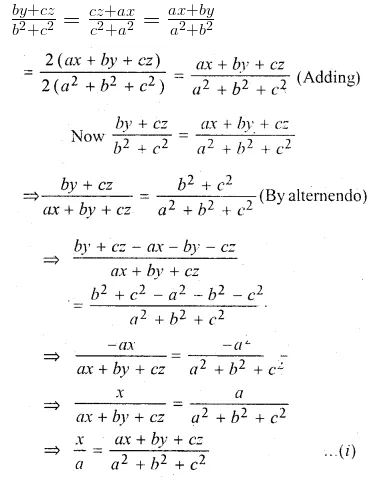# ML Aggarwal Class 10 Solutions for ICSE Maths Chapter 7 Ratio and Proportion Chapter Test

## ML Aggarwal Class 10 Solutions for ICSE Maths Chapter 7 Ratio and Proportion Chapter Test

ML Aggarwal Class 10 Solutions for ICSE Maths Chapter 7 Ratio and Proportion Chapter Test

Question 1.
Find the compound ratio of:
(a + b)2 : (a – b )2
(a2 – b2) : (a2 + b2)
(a4 – b4) : (a + b)4
Solution:Question 2.
If (7p + 3q) : (3p – 2q) = 43 : 2 find p : q
Solution:Question 3.
If a : b = 3 : 5, find (3a + 5b) : (7a – 2b).
Solution:Question 4.
The ratio of the shorter sides of a right-angled triangle is 5 : 12. If the perimeter of the triangle is 360 cm, find the length of the longest side.
Solution:Question 5.
The ratio of the pocket money saved by Lokesh and his sister is 5 : 6. If the sister saves Rs 30 more, how much more the brother should save in order to keep the ratio of their savings unchanged?
Solution:Question 6.
In an examination, the number of those who passed and the number of those who failed were in the ratio of 3 : 1. Had 8 more appeared, and 6 less passed, the ratio of passed to failures would have been 2 : 1. Find the number of candidates who appeared.
Solution:Question 7.
What number must be added to each of the numbers 15, 17, 34 and 38 to make them proportional?
Solution:Question 8.
If (a + 2b + c), (a – c) and (a – 2b + c) are in continued proportion, prove that b is the mean proportional between a and c.
Solution:Question 9.
If 2, 6, p, 54 and q are in continued proportion, find the values of p and q.
Solution:Question 10.
If a, b, c, d, e are in continued proportion, prove that: a : e = a4 : b4.
Solution: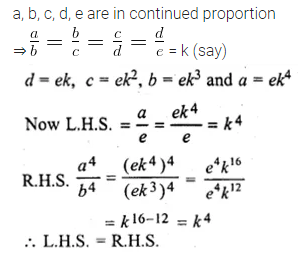Question 11.
Find two numbers whose mean proportional is 16 and the third proportional is 128.
Solution: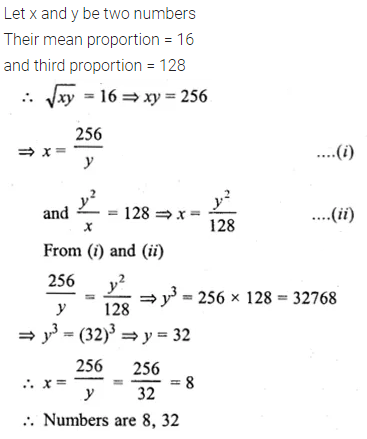Question 12.
If q is the mean proportional between p and r, prove that:
$${ p }^{ 2 }-{ 3q }^{ 2 }+{ r }^{ 2 }={ q }^{ 4 }\left( \frac { 1 }{ { p }^{ 2 } } -\frac { 3 }{ { q }^{ 2 } } +\frac { 1 }{ { r }^{ 2 } } \right)$$
Solution: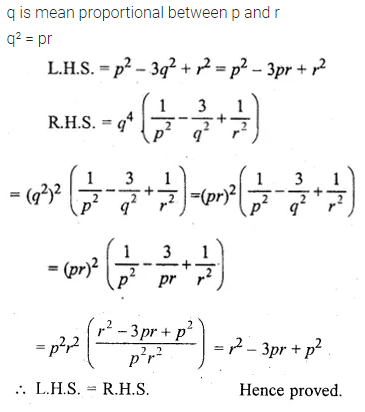Question 13.
If $$\frac { a }{ b } = \frac { c }{ d } = \frac { e }{ f }$$, prove that each ratio is
(i) $$\sqrt { \frac { { 3a }^{ 2 }-{ 5c }^{ 2 }+{ 7e }^{ 2 } }{ { 3b }^{ 2 }-{ 5d }^{ 2 }+{ 7f }^{ 2 } } }$$
(ii) $${ \left[ \frac { { 2a }^{ 3 }+{ 5c }^{ 3 }+{ 7e }^{ 3 } }{ { 2b }^{ 3 }+{ 5d }^{ 3 }+{ 7f }^{ 3 } } \right] }^{ \frac { 1 }{ 3 } }$$
Solution: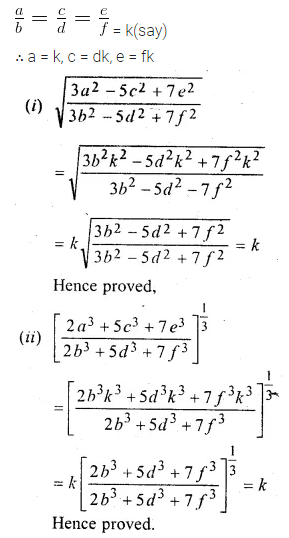Question 14.
If $$\frac { x }{ a } = \frac { y }{ b } = \frac { z }{ c }$$, prove that
$$\frac { { 3x }^{ 3 }-{ 5y }^{ 3 }+{ 4z }^{ 3 } }{ { 3a }^{ 3 }-{ 5b }^{ 3 }+{ 4c }^{ 3 } } ={ \left( \frac { 3x-5y+4z }{ 3a-5b+4c } \right) }^{ 3 }$$
Solution:Question 15.
If x : a = y : b, prove that
$$\frac { { x }^{ 4 }+{ a }^{ 4 } }{ { x }^{ 3 }+{ a }^{ 3 } } +\frac { { y }^{ 4 }+{ b }^{ 4 } }{ { y }^{ 3 }+{ b }^{ 3 } } =\frac { { \left( x+y \right) }^{ 4 }+{ \left( a+b \right) }^{ 4 } }{ { \left( x+y \right) }^{ 3 }+{ \left( a+b \right) }^{ 3 } }$$
Solution: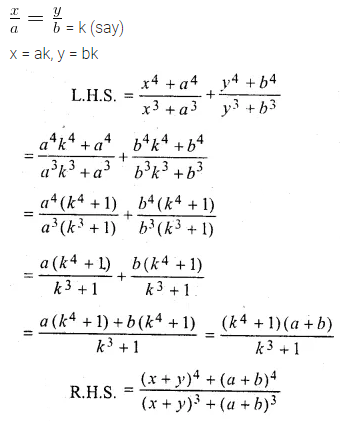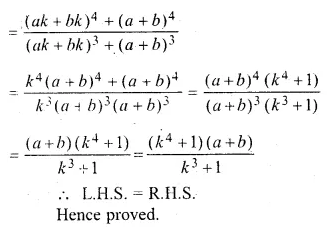Question 16.
If $$\frac { x }{ b+c-a } =\frac { y }{ c+a-b } =\frac { z }{ a+b-c }$$ prove that each ratio’s equal to :
$$\frac { x+y+z }{ a+b+c }$$
Solution:Question 17.
If a : b = 9 : 10, find the value of
(i) $$\frac { 5a+3b }{ 5a-3b }$$
(ii) $$\frac { { 2a }^{ 2 }-{ 3b }^{ 2 } }{ { 2a }^{ 2 }+{ 3b }^{ 2 } }$$
Solution: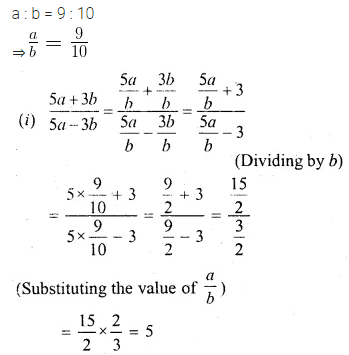Question 18.
If (3x² + 2y²) : (3x² – 2y²) = 11 : 9, find the value of $$\frac { { 3x }^{ 4 }+{ 25y }^{ 4 } }{ { 3x }^{ 4 }-{ 25y }^{ 4 } }$$ ;
Solution:Question 19.
If $$x=\frac { 2mab }{ a+b }$$ , find the value of
$$\frac { x+ma }{ x-ma } +\frac { x+mb }{ x-mb }$$
Solution:Question 20.
If $$x=\frac { pab }{ a+b }$$ ,prove that $$\frac { x+pa }{ x-pa } -\frac { x+pb }{ x-pb } =\frac { 2\left( { a }^{ 2 }-{ b }^{ 2 } \right) }{ ab }$$
Solution:Question 21.
Find x from the equation $$\frac { a+x+\sqrt { { a }^{ 2 }-{ x }^{ 2 } } }{ a+x-\sqrt { { a }^{ 2 }-{ x }^{ 2 } } } =\frac { b }{ x }$$
Solution: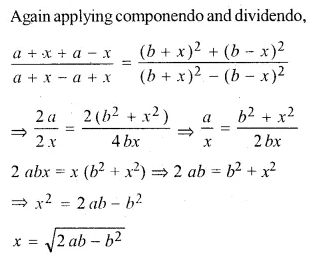Question 22.
If $$x=\frac { \sqrt [ 3 ]{ a+1 } +\sqrt [ 3 ]{ a-1 } }{ \sqrt [ 3 ]{ a+1 } -\sqrt [ 3 ]{ a-1 } }$$, prove that :
x³ – 3ax² + 3x – a = 0
Solution:Question 23.
If $$\frac { by+cz }{ b^{ 2 }+{ c }^{ 2 } } =\frac { cz+ax }{ { c }^{ 2 }+{ a }^{ 2 } } =\frac { ax+by }{ { a }^{ 2 }+{ b }^{ 2 } }$$, prove that each of these ratio is equal to $$\frac { x }{ a } =\frac { y }{ b } =\frac { z }{ c }$$
Solution: